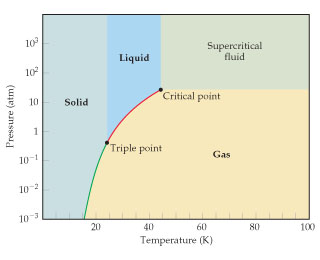# Problem: Use the phase diagram for neon to answer the following questions:What is the approximate value of the normal melting point?

###### FREE Expert Solution
• Melting point of a substance is the temperature where solid changes to liquid. At melting point, solid and liquid exists in equilibrium
• When it says at normal boiling pointthis is the temperature when the change of solid to liquid happens at 1 atm.###### Problem Details

Use the phase diagram for neon to answer the following questions:What is the approximate value of the normal melting point?